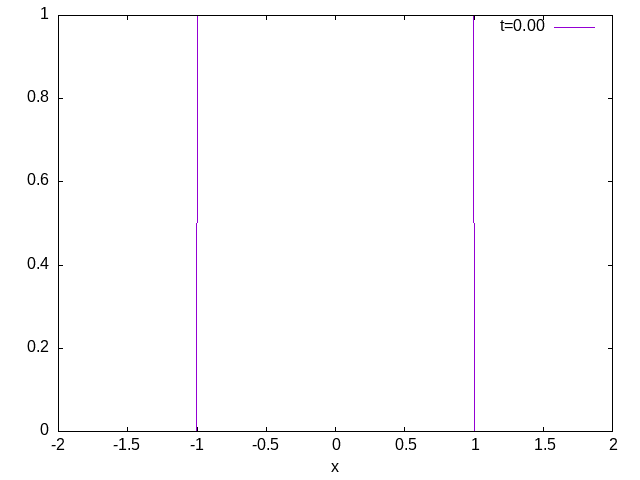# collapse of a rectangular visco-plastic Herschel-Bulkley fluid

Explicit resolution of 1D collapse of Herschel-Bulkley fluid \displaystyle \frac{\partial h}{\partial t}+ \frac{\partial Q(h)}{\partial x}=0, \displaystyle Q= \Bigg( \frac{n Y^{1+1/n}}{(1+n)(1+2n) }((2 n+1) h- n Y) (S - \frac{\partial h}{\partial x }) \left( | S - \frac{\partial h}{\partial x}|\right)^{\frac{1}{n}-1} \Bigg) with \displaystyle Y = max( h -\frac{B}{| S- \frac{\partial h}{\partial x } | } ,0).

set size ratio .3
set samples 9
set label "h i-1" at 1.5,3.1
set label "h i" at 2.5,3.15
set label "h i+1" at 3.5,2.5
set xtics ("i-2" 0.5, "i-1" 1.5, "i" 2.5,"i+1" 3.5,"i+2" 4.5,"i+3" 5.5)
set arrow from 2,1 to 2.5,1
set arrow from 3,1 to 3.5,1
set label "Q i" at 2.1,1.25
set label "Q i+1" at 3.1,1.25

set label "x i-1/2" at 1.5,0.25
set label "x i" at 2.4,0.25
set label "x i+1/2" at 3.,0.25

set label "x"  at 0.5,2+sin(0)
set label "x"  at 1.5,2+sin(1)
set label "x"  at 2.5,2+sin(2)
set label "x"  at 3.5,2+sin(3)
set label "x"  at 4.5,2+sin(4)
f(x) = (2+sin(x))*(x<=4)
p[-1:7][0:4] f(x) w steps not,f(x) w impulse not linec 1(script)

## Code

#include "grid/cartesian1D.h"
#include "run.h"

scalar h[];
scalar Y[];
scalar Q[];
scalar hp[],hpc[],nu[];
scalar dQ[];
double n,dt,S,B,tmax,inct=0.0001;
char s;

int main() {
L0 = 4;
B = .25 ;
X0 = -L0/2;
S = 0.000000001;
N = 512;
DT = (L0/N)*(L0/N)*1.0;
n = 1.3;  // n=1 Bingham pur
tmax = 500;

{
sprintf (s, "x.txt");
FILE * fp = fopen (s, "w");
fclose(fp);
sprintf (s, "shape.txt");
FILE * fs = fopen (s, "w");
fclose(fs);
inct = DT;
run();
}
}
event init (t = 0) {
foreach(){
h[] =1*(fabs(x)<1) ;
Q[]=0;
}
boundary ({h,Q});
}

event output (t += inct; t < tmax) {
double  xf=0,xe=0;
inct = inct*2;
inct = min(1,inct);
foreach(){
xf = h[] > 1e-4 ?  max(xf,x) :  xf ;
xe = h[] > 1e-4 ?  min(xe,x) :  xe ;
}

sprintf (s, "front.txt");
FILE * f = fopen (s, "w");
foreach()
fprintf (f, "%g %g %g \n", fmin((x-xf),0), h[], xe-xf);
fclose(f);
fprintf (stderr, "%g %g %g \n", t, xf, xe);
sprintf (s, "x.txt");
FILE * fp = fopen (s, "a");
fprintf (fp, "%g %g  \n", t, xf);
fclose(fp);
}

event printdata (t += 0.25, t < tmax){
sprintf (s, "shape.txt");
FILE * fp = fopen (s, "a");
foreach()
fprintf (fp, "%g %g %g %g %g \n", x, h[], Q[], Y[], t);
fprintf (fp, "\n");
fclose(fp);
}

event integration (i++) {
double dt = DT;

dt = dtnext (dt);

foreach()
hp[] =  ( h[0,0] - h[-1,0] )/Delta;
boundary ({hp});

foreach()
hpc[] =  ( h[1,0] - h[-1,0] )/2/Delta;
boundary ({hp});

foreach()
Y[] =  max( h[] - B/fabs(S-hpc[])  ,0);
boundary ({Y});

foreach()
nu[] =  n * ( pow(Y[-1,0],((n+1)/n))*((2*n+1)*h[-1,0] - n*Y[-1,0])/((1+n)*(2*n+1)) +
pow(Y[0,0] ,((n+1)/n))*((2*n+1)*h[0,0]  - n*Y[0,0] )/((1+n)*(2*n+1)) )/2 ;
boundary ({nu});

foreach()
Q[] =  nu[] *  pow(fabs( S-hp[] ),1/n-1) * (S-hp[]) ;
boundary ({Q});

foreach()
dQ[] =  ( Q[1,0] - Q[0,0] )/Delta;
boundary ({dQ});

foreach(){
h[] +=  - dt*dQ[];
}
boundary ({h});
}

## Run

Then compile and run, either by hand either with make:

qcc  -g -O2 herschel-column-noSV.c -o herschel-column-noSV
./herschel-column-noSV > out

make herschel-column-noSV.tst;make herschel-column-noSV/plots
make herschel-column-noSV.c.html ; open herschel-column-noSV.c.html

source ../c2html.sh herschel-column-noSV

## Results

Playing here with gnuplot and every :

every I:J:K:L:M:N

I Line increment/ J Data block increment/ K The first line/ L The first data block/ M The last line/ N The last data block

ex:


every ::3 skip the first 3 lines
every ::3::5  plot from the 4-th to 6-th lines
every ::0::0  plot the first line only
every 2::::6  plot the 1,3,5,7-th lines
every :2  plot every 2 data block
every :::5::8 plot from 5-th to 8-th data blocks

p'shape.txt' ev :20::0::100  // plot from  0 to 100th every 20

plot the first and last shapes :

  reset
B=.25;S=0;xf=(9./8/B*(1+S*S/2)**2)**(1./3)
set xlabel "x"
set ylabel "h(x,0),h(x,infinity)"
stats 'shape.txt' u 1;
k = (STATS_records/512)-1
p[][0:1.5]'shape.txt' every :::k::k t'last' w lp,'' every :::0::0 t 'init' w l ,sqrt(2*B*(xf-x)) not ,sqrt(2*B*(xf+x))  not(script)

front as function of time

  reset
B=.25;S=0;xf=(9./8/B*(1+S*S/2)**2)**(1./3)
set logscale x
set xlabel "t"
set ylabel "x"
set key bottom
p'x.txt'  t'cul' w l,xf  t'analytic'(script)

gnuplot generates a gif:

reset
set xlabel "x"
!echo "p[:][:1]'shape.txt' ev :::k::k title sprintf(\"t=%.2f\",k*.05) w l;k=k+1 ;if(k<200) reread " > mov.gnu
set term gif animate;
set output 'slump.gif'
k=0
l'mov.gnu'(script)

Gif animated of the slump (reload to refresh) or click on image for animation:a gif